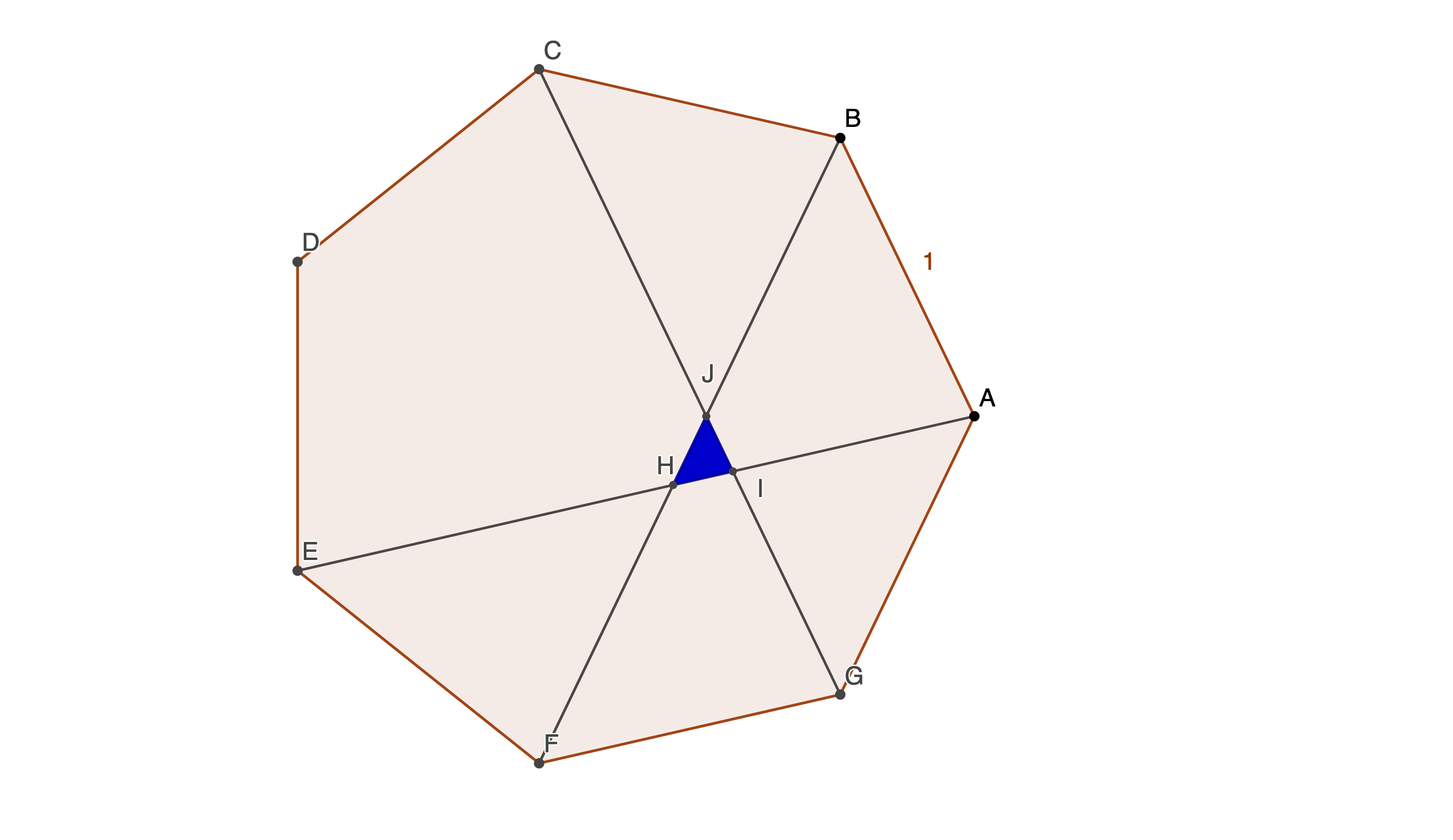# Septic Triangle

Geometry Level 5The figure shows a regular septagon with side length 1. $\triangle HIJ$ is formed by diagonals $AE, BF$, and $CG$. What is the area of $\triangle HIJ$? Find a closed-form solution, convert it to a decimal number, and submit the sum of the first 50 digits to the right of the decimal point.

×